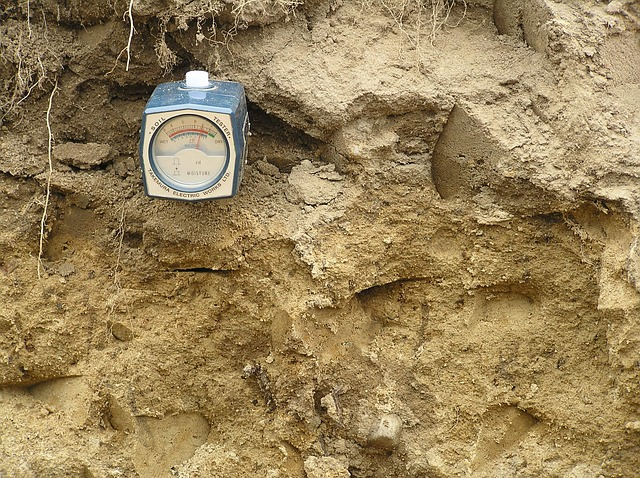Feb 26, 2016

Feb 26, 2016

Feb 26, 2016

# Experiment To Find PH Value of Given Water Sample## Theory:

"PH" value is the measure of concentration of hydrogen in water it shows the alkanity or acidity of water. Mathematically PH may be defined as:

The negative log of hydrogen ion concentration

PH - log [H]

Sorenson in 1909 introduced this scale for the first time.

H20 <--> H4 + OH

This reaction shows that the number of H4 and OH ions are equal experimentally it has been proved that the product of concentration of H4 and OH is a constant quality K , whose value was found to be 10 - 14 i.e

[H4][OH = K--> [H4][OH] -10
Log [H4] + Log [OH] = -14

--> - Log [H4] - Log [OH] = 14
-->ph 4 poh =14

But for what pH = POH
2PH = 14-->pH = 7

for acids PH ranges from 1 to 7 and for base PH ranges from8 to 14 There Are Two methods to determine the PH values of given water sample,

1. Colorimetric method
2. Electrometric method

### Importance of pH:

PH is very important in the control of number of water and waste water treatment processes and in the control of corrosion.

### W.H.O guide line value:

World organization suggested a guideline value of (6.5) to (8.5) for pH of water.

## Apparatus & Chemicals:

Buffers (pH4,pH) standard pH solution problem pH meter stand and colorimetric paper and water sample

## Procedure:

### 1. Colorimetric Method:

Dip the colorimetric paper in water sample. Compute the color of paper with color from the table and note the PH of water against this color, This is the PH of the sample.

### 2. Electrometric Method:

1. Press "01" key of PH meter to bring the meter in working condition.
2. Press the PH key and calibrate key so that the screen shows "00.00" reading.
3. Dip the problem into standard solution of PH - 7 and press "standard" key so that the screen gives 7.00 reading.
4. Dip the probe in water sample and press"disperser" key and PH key to get the PH of the sample.
5. Read the value of PH from Screen.